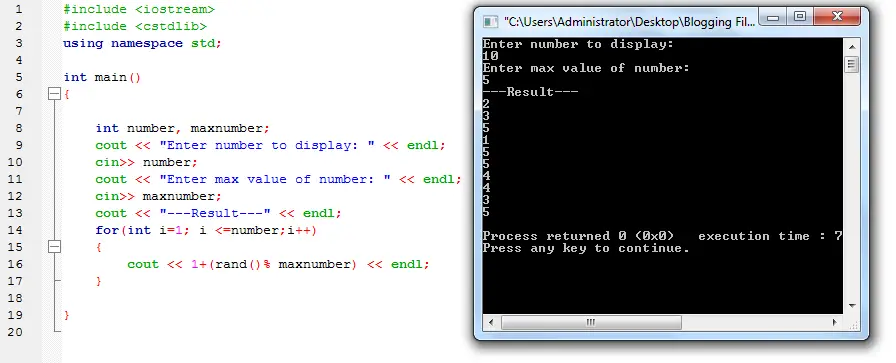# Random Numbers in C++• Version
• File Size 1.32 KB
• File Count 1
• Create Date May 9, 2016
• Last Updated May 9, 2016

# Random Numbers in C++

Random Numbers in C++

A program in c++ that generates random numbers using rand function. The user will need to input how many numbers he/she want to display and the max value of the number.

The numbers entered will be then executed inside a for loop and random numbers will displayed.
Happy Coding!

Source code:

```#include < iostream >
#include < cstdlib >
using namespace std;

int main()
{

int number, maxnumber;
cout << "Enter number to display: " << endl;
cin >> number;
cout << "Enter max value of number: " << endl;
cin >> maxnumber;
cout << "---Result---" << endl;
for(int i=1; i <= number; i++)
{
cout <<  1 + (rand()% maxnumber)  << endl;
}

}
```